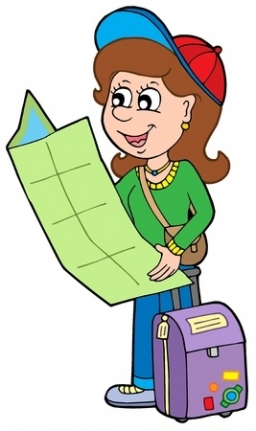# The tourist

The tourist has walked 3/4 of the route. The destination is 12.5 km away. How many kilometers did the route measure?

Correct result:

x =  50 km

#### Solution:

1/4x = 12.5

1/4•x = 12.5

x = 50

x = 50

Calculated by our simple equation calculator.We would be very happy if you find an error in the example, spelling mistakes, or inaccuracies, and please send it to us. We thank you!Tips to related online calculators
Need help calculate sum, simplify or multiply fractions? Try our fraction calculator.
Do you have a linear equation or system of equations and looking for its solution? Or do you have quadratic equation?
Do you want to convert length units?

## Next similar math problems:

• Lengths of the poolMiguel swam 6 lengths of the pool. Mat swam 3 times as far as Miguel. Lionel swam 1/3 as far as Miguel. How many lengths did mat swim?
• TeacherTeacher Rem bought 360 pieces of cupcakes for the outreach program of their school. 5/9 of the cupcakes were chocolate flavor and 1/4 wete pandan flavor and the rest were a vanilla flavor. How much more pandan flavor cupcakes than vanilla flavor?
• Athletic raceBefore a race, you start 4 5/8 feet behind your friend. At the halfway point, you are 3 2/3 feet ahead of your friend. What is the change in distance between you and your friend from the beginning of the race?
• In fractionsAn ant climbs 2/5 of the pole on the first hour and climbs 1/4 of the pole on the next hour. What part of the pole does the ant climb in two hours?
• NormThree workers planted 3555 seedlings of tomatoes in one dey. First worked at the standard norm, the second planted 120 seedlings more and the third 135 seedlings more than the first worker. How many seedlings were standard norm?
• Trees3/5 trees are apples, cherries are 1/3. 5 trees are pear. How many is the total number of trees?
• TreesFrom the total number of trees in the orchard, there are two-fifths pearls and apples are three eighty. The rest of the trees are 9 ceremonial. How many trees are in the set?
• ClassroomOne-eighth of 9th class was interested in studying at a grammar school, at a business academy one sixth, at secondary vocational schools quarter, to SOU one third and the remaining three students were interested in the school of art direction. How many st
• Passenger boatTwo-fifths of the passengers in the passenger boat were boys. 1/3 of them were girls and the rest were adult. If there were 60 passengers in the boat, how many more boys than adult were there?
• Dropped sheetsThree consecutive sheets dropped from the book. The sum of the numbers on the pages of the dropped sheets is 273. What number has the last page of the dropped sheets?
• Simple equation 8Solve the following equation: 36=-(1+7x)-6(-7-x)
• Unknown number 6Determine x if 1/6 of x is equal to 2/5 of the number 24.
• EquationFind x: x + 1/2 = 1/3
• Fraction and a decimalWrite as a fraction and a decimal. One and two plus three and five hundredths
• Write 2Write 791 thousandths as fraction in expanded form.
• Eq-fracSolve the following equation with fractions: h + 1/3 =5/3
• Find xSolve: if 2(x-1)=14, then x= (solve an equation with one unknown)